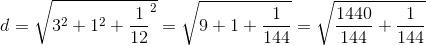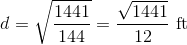## Example Questions

### Example Question #1 : How To Find The Diagonal Of A Prism

A right, rectangular prism has has a length of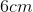, a width of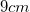, and a height of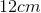. What is the length of the diagonal of the prism?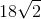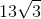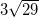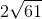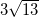Explanation:

First we must find the diagonal of the prism's base (). This can be done by using the Pythagorean Theorem with the length () and width (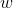):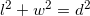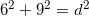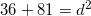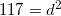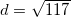Therefore, the diagonal of the prism's base is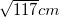. We can then use this again in the Pythagorean Theorem, along with the height of the prism (), to find the diagonal of the prism ():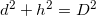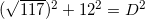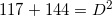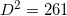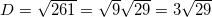Therefore, the length of the prism's diagonal is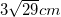.

### Example Question #2 : How To Find The Diagonal Of A Prism

What is the diagonal of a rectangular prism with a height of 4, width of 4 and height of 6?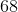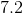Cannot be determined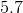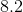Explanation:

In order to solve this problem, it's helpful to visualize where the diagonal is within the prism.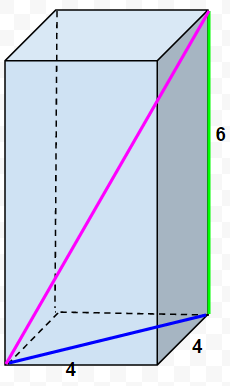In this image, the diagonal is the pink line. By noting how it relates to the blue and green lines, we can observe how the pink line is connected and creates a right triangle. This very quickly becomes a problem that employs the Pythagorean theorem.

The goal is essentially to find the hypotenuse of this sketched-in right triangle; however, only one of the legs is given: the green line, the height of the prism. The blue line can be solved for by understanding that it is the measurement of the diagonal of a 4x4 square.

Either using trig functions or the rules for a special 45/45/90 triangle, the blue line measures out to be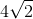The rules for a 45/45/90 triangle: both legs are "" and the hypotenuse is "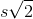". Keep in mind, this is is only for isosceles right triangles.

Now that both legs are known, we can solve for the hypotenuse (diagonal).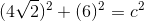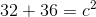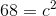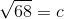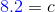### Example Question #3 : How To Find The Diagonal Of A Prism

Find the diagonal of a right rectangular prism if the length, width, and height are 3,4, and 5, respectively.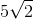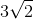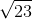Explanation:

Write the diagonal formula for a rectangular prism.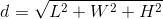Substitute and solve for the diagonal.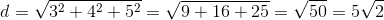### Example Question #4 : How To Find The Diagonal Of A Prism

If the dimensions of a right rectangular prism are 1 yard by 1 foot by 1 inch, what is the diagonal in feet?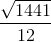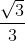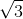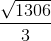Explanation:

Convert the dimensions into feet.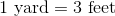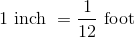The new dimensions of rectangular prism in feet are: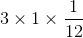Write the formula for the diagonal of a right rectangular prism and substitute.